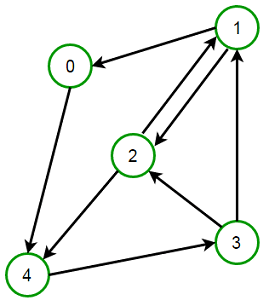# Check if Graph is Strongly Connected or not using one DFS Traversal

Given a directed graph, check if it is strongly connected or not. A directed graphs is said to be strongly connected if every vertex is reachable from every other vertex.

For example,

Below graph is strongly connected as path exists between all pairs of vertices.Prerequisite:

In previous post, we have discussed a solution for that requires two DFS traversals of a Graph. We can check if graph is strongly connected or not by doing only one DFS traversal of the graph.

When we do a DFS from a vertex v in an directed graph, there could be many edges going out of its sub tree. When we say subtree rooted at v, we mean all v’s descendants including the vertex itself. The edges which are going out of the sub tree will either be a back edge or a cross edge. Forward edge cannot be going out of the sub tree as they can only be coming in to the sub tree or if it starts from within the sub tree it will go within the sub tree only.

We can say that the graph is strongly connected if and only if for every edge u->v in the graph, there is at-least one back-edge or cross-edge that is going out of subtree rooted at v.

##### How should we modify DFS so that we can check if there is a out-edge going out of every sub tree?

We can modify DFS such that DFS(v) returns the smallest arrival time to which there is an out-edge from the sub tree rooted at v. For example, let arrival(v) be the arrival time of vertex v in the DFS. Then if there is a edge out of the sub tree rooted at v, it’s to something visited before v & therefore with a smaller arrival value.

Remember for a back edge or cross edge u -> v,arrival[u] > arrival[v]

Suppose there are four edges going out of sub-tree rooted at v to vertex a, b, c and d and with arrival time arrival(a), arrival(b), arrival(c) and arrival(d) respectively. We look at their four arrival times & consider the smallest among them and that will be the value returned by DFS(v). i.e. DFS(v) returns min of arrival(a), arrival(b), arrival(c) and arrival(d). But before returning, we have to check that min(arrival(a), arrival(b), arrival(c), arrival(d)) is less than the arrival(v). If min(arrival(a), arrival(b), arrival(c), arrival(d)) is less than the arrival(v), then that means that at-least one back-edge or cross edge is going out of the sub tree rooted at v. If not, then we can stop the procedure and say that the graph is not strongly connected.

This is demonstrated below in C++:

Output:

Graph is Strongly Connected

The time complexity of above solutions is O(n + m) where n is number of vertices and m is number of edges in the graph. Please note that O(m) may vary between O(1) and O(n2), depending on how dense the graph is.(1 votes, average: 5.00 out of 5)Loading...

Please use our online compiler to post code in comments. To contribute, get in touch with us.
Like us? Please spread the word and help us grow. Happy coding 🙂